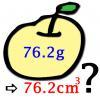#### You may also likeA 1 metre cube has one face on the ground and one face against a wall. A 4 metre ladder leans against the wall and just touches the cube. How high is the top of the ladder above the ground?### Archimedes and Numerical Roots

The problem is how did Archimedes calculate the lengths of the sides of the polygons which needed him to be able to calculate square roots?### More or Less?

Are these estimates of physical quantities accurate?

# Time to Evolve

### Why do this problem?

This task will give practice in making sensible estimates, approximations and making a prediction based on a range of possible values. Although the content is relatively simple, answers could be given to this question at a range of levels and the problem would suit both Stage 3 and Stage 5 students as an exercise in thinking.

### Possible approach

There are various ways in which approximations can be made and it might be good to let students attempt to make their own approximations before opening up to group discussion. As they work on the problem, various decisions will need to be made and various uncertainties will arise (for example: at what stage between maturity and death of a parent was a particular offspring in the chain of descendants produced?). Students should be encouraged to take notes of these points, as discussion of these forms a key part of the evaluation process.

It is interesting to ask students first to give a guess/estimate of the number of descendants without doing any calculation. Students could then work on the problem in pairs to come up with an estimate and then share their ideas with other groups. Whose estimations seem best? Can students justify clearly their estimations to each other? Do any students wish to revise their estimates in light of such interaction?

Once estimations have been constructed and discussed it would be worth relating the estimates back to the original question: how reliable might we consider our estimates of the number of generations to be? What questions would this raise? What information might lead to better estimates?

Finally, how did the students' initial guesses/estimates fit in with the calculated estimates? This allows students to reflect on the size of the final answers. Were they much larger or smaller than expected?

### Key questions

Key questions should be used as appropriate to help students find their way into the question:
• Can you make a start to the question by giving a very rough estimate of the numbers that go into the table? How could you use these numbers to work out the number of generations?
• When you fill in the table, what sensible range of choices do you have for each entry?
• When you calculate the number of descendants from a filled-in table, what uncertainties are there?
• How could you use a filled-in table to calculate the maximum or minimum number of descendants?

### Possible extension

The fullest solution to this task/main extension involves a careful investigation of the minimum and maximum possible number of descendants. For those with a knowledge of statistics, you might refer them to Time to Evolve 2.

As a very general extension discussion point, it is worth mentioning that the evolution from one species to another occurs through gene mutation. Can students get any sense of the rate at which the genes would have to mutate to account for the required genetic change over the given time period? This activitiy would, of course, be research intensive.

### Possible support

Some students might feel uncomfortable that there are no 'right' numbers to fill into the table and no 'right' answer to the estimation question. Encourage them that it is OK to make a rough estimate to begin with and then to refine that estimate later. Give them some concrete estimates of time to maturity/death if necessary.